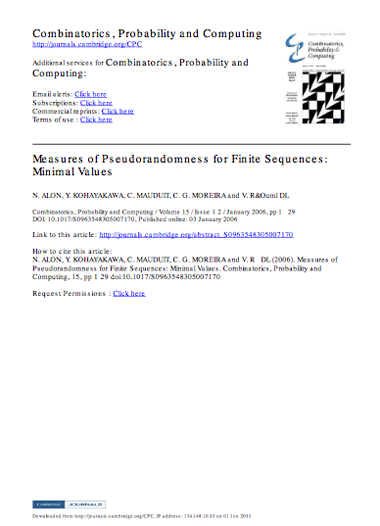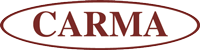# Walking on Real Numbers

## Publications

Summary of Publication Publication Preview

#### Walking on Real Numbers - CORE PUBLICATION

Francisco J. Aragón Artacho, David H. Bailey, Jonathan M. Borwein and Peter B. Borwein

(Math. Intelligencer 35 (1) (July 2013), 42—60)

This is the core publication of our research into real numbers.
Full Size Version // Reduced Size Version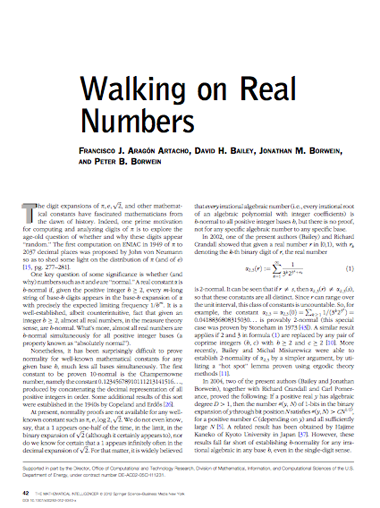#### Normal Numbers and Normality Measure

Christoph Aistleitner

(Combinatorics, Probability and Computing 22 (3) (May 2013), 342—345)

In a paper published in this journal, Alon, Kohayakawa, Mauduit, Moreira and Rödl proved that the minimal possible value of the normality measure of an N-element binary sequence satisfies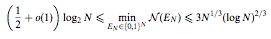for sufficiently large N, and conjectured that the lower bound can be improved to some power of N. In this note it is observed that a construction of Levin of a normal number having small discrepancy gives a construction of a binary sequence E N with (E N ) = O((log N)2), thus disproving the conjecture above.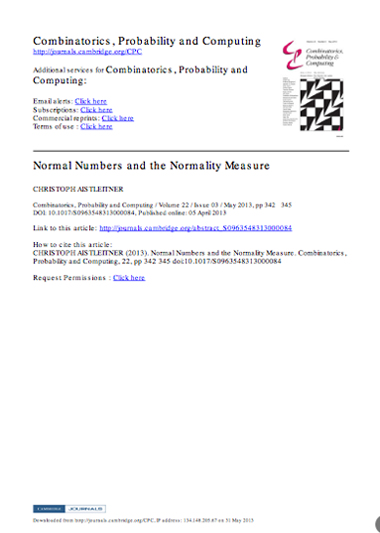#### Mahler measures, short walks and log-sine integrals

Jonathan M. Borwein and Armin Straub

(Theoretical Computer Science 479 (1) (April 2013), 4–21)

The Mahler measure of a polynomial in several variables has been a subject of much study over the past thirty years — very few closed forms are proven but more are conjectured. In the case of multiple Mahler measures more tractable but interesting families exist. Using values of log-sine integrals we provide systematic evaluations of various higher and multiple Mahler measures.

The evaluations in terms of log-sine integrals become particularly useful in light of the fact that log-sine integrals may be automatically reexpressed as polylogarithmic values. We present this correspondence along with related generating functions for log-sine integrals.

Our initial interest in considering Mahler measures stems from a study of uniform random walks in the plane as first introduced by Pearson. The main results on the moments of the distance traveled by an nn-step walk, as well as the corresponding probability density functions, are reviewed. It is the derivative values of the moments that are Mahler measures.

This work would be impossible without very extensive symbolic and numeric computations. It also makes frequent use of the new NIST Handbook of Mathematical Functions and similar tools. Our intention is to show off the interplay between numeric and symbolic computing while exploring the three mathematical topics in the title.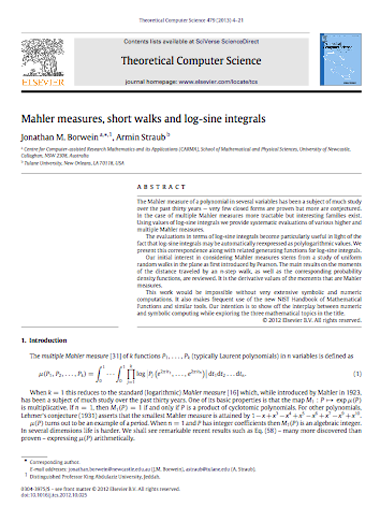#### Closed Forms: What They Are and Why We Care

Jonathan M. Borwein and Richard E. Crandall

(Notices of the AMS 60 (1) (January 2013), 50—65)

Mathematics abounds in terms that are in frequent use yet are rarely made precise. Two such are rigorous proof and closed form (absent the technical use within differential algebra). If a rigorous proof is “that which ‘convinces’ the appropriate audience,” then a closed form is “that which looks ‘fundamental’ to the requisite consumer.” In both cases, this is a community-varying and epoch-dependent notion. What was a compelling proof in 1810 may well not be now; what is a fine closed form in 2010 may have been anathema a century ago. In this article we are intentionally informal as befits a topic that intrinsically has no one “right” answer. Let us begin by sampling the Web for various approaches to informal definitions of “closed form”.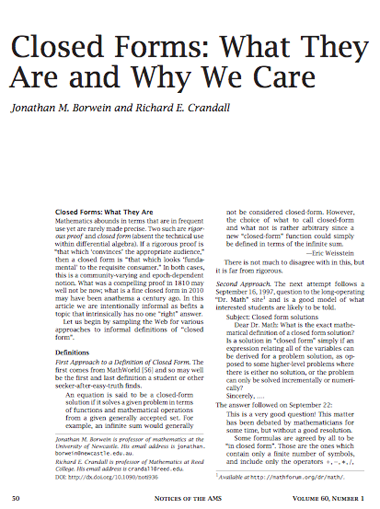#### Être normal? Pas si facile!

Jean-Paul Delahaye

(Pour la Science 422 (December 2012))

In 1908, the French mathematician Émile Borel (1871-1956) queries on particular properties that may have the usual numbers decimal, such as √2, e or π. He introduced the concept of "normal number" which we will discuss below the definition.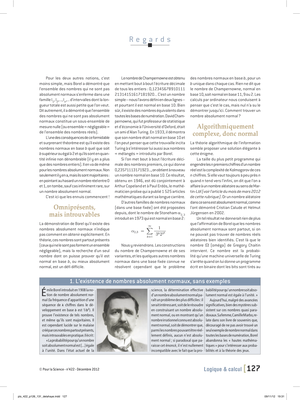#### The Life of Pi

Jonathan M. Borwein

(Prepared for Berggren Festschrift, Draft VIII. 19/06/2012)

The desire to understand π the challenge, and originally the need, to calculate ever more accurate values of π the ratio of the circumference of a circle to its diameter, has challenged mathematicians–great and less great — for many centuries. It has also, especially recently, provided compelling examples of computational mathematics. π uniquely in mathematics, is pervasive in popular culture and the popular imagination.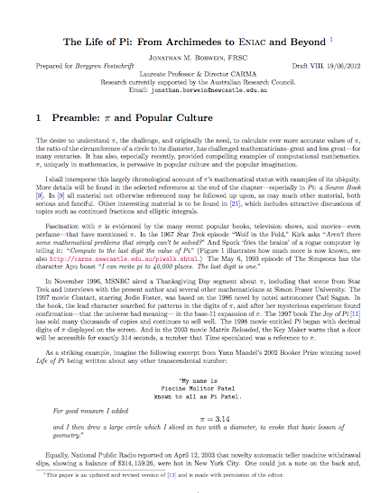#### Abnormality of Stoneham Numbers

David H. Bailey and Jonathan M. Borwein

(The Ramanujan Journal 29 (1-3) (June 2012), 409-422)

This paper examines “Stoneham constants,” namely real numbers of the form α b,c= n11/(c nb c n) , for coprime integers b≥2 and c≥2. These are of interest because, according to previous studies, α b,c is known to be b-normal, meaning that every m-long string of base-b digits appears in the base-b expansion of the constant with precisely the limiting frequency bm .

So, for example, the constant α 2,3= n11/(3 n2 3 n) is 2-normal. More recently it was established that α b,c is not bc-normal, so, for example, α 2,3 is provably not 6-normal. In this paper, we extend these findings by showing that α b,c is not B-normal, where B=b p c q r, for integers b and c as above, p,q,r≥1, neither b nor c divide r, and the condition D=c q/p r 1/p /b c−1<1 is satisfied. It is not known whether or not this is a complete catalog of bases to which α b,c is nonnormal. We also show that the sum of two B-nonnormal Stoneham constants as defined above, subject to some restrictions, is B-nonnormal.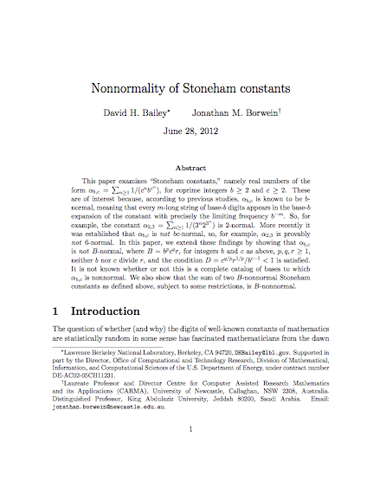#### Proof of some Conjectured Formulas for π

Tewodros Amdeberhan, David Borwein, Jonathan M. Borwein and Armin Straub

(Journal of Mathematical Physics 53 (7) (May 2012))

In a recent study of representing Dirac's delta distribution using q-exponentials, M. Jauregui and C. Tsallis experimentally discovered formulae for π as hyper-geometric series as well as certain integrals. Herein, we offer rigorous proofs of these identities using various methods and our primary intent is to lay down an illustration of the many technical underpinnings of such evaluations.

This includes an explicit discussion of creative telescoping and Carlson's Theorem. We also generalize the Jauregui-Tsallis identities to integrals involving Chebyshev polynomials. In our pursuit, we provide an interesting tour through various topics from classical analysis to the theory of special functions.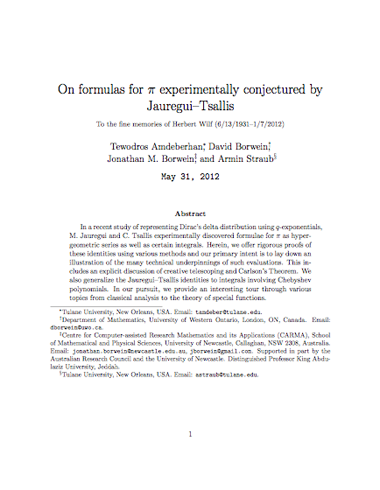#### Ramanujan and π

Jonathan M. Borwein

(May 2012)

This contribution highlights the progress made regarding Ramanujan's work on Pi since the centennial of his birth in 1987.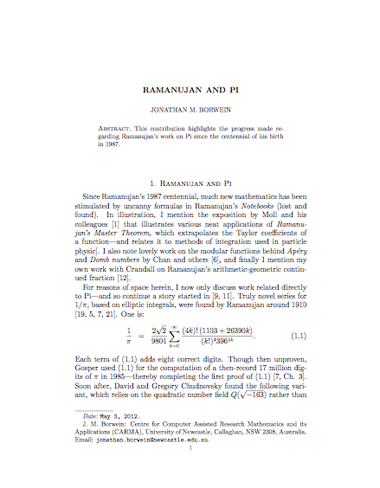#### The art of π, φ and e

Martin Krzywinski

(mkweb.bcgsc.ca (April 2012))

Numerology is bogus, but art based on numbers has a beautiful random quality. A visually beautiful article.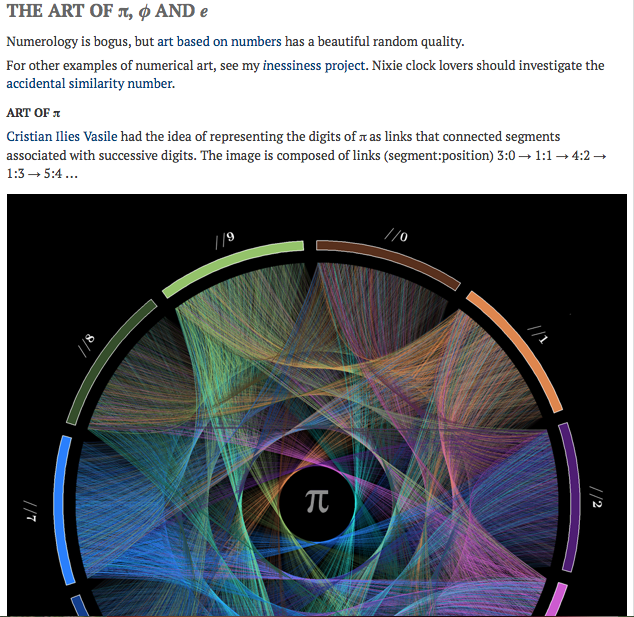#### On the Normality of Pi

David H. Bailey, Jonathan M. Borwein, Cristian S. Calude, Michael J. Dineen, Monica Dumitrescu and Alex Yee

(Experimental Mathematics 21 (4) (February 2012), 375—384)

Using the results of several extremely large recent computations, we tested positively the normality of a prefix of roughly four trillion hexadecimal digits of π.. This result was used by a Poisson process model of normality of π.: in this model, it is extraordinarily unlikely that π. is not asymptotically normal base 16, given the normality of its initial segment.

Extended Version of the Paper HERE (PDF)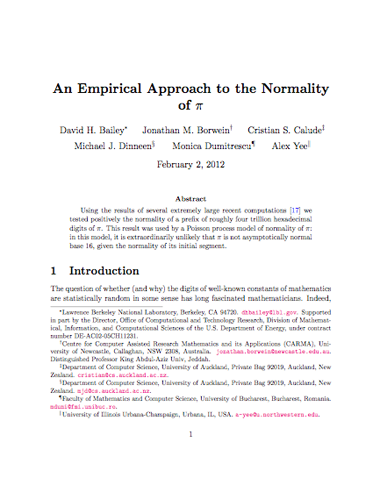#### Mahler measures, short walks and log-sine integrals

Jonathan M. Borwein and Armin Straub

(January 2012)

The Mahler measure of a polynomial in several variables has been a subject of much study over the past thirty years — very few closed forms are proven but more are conjectured. In the case of multiple Mahler measures more tractable but interesting families exist. Using values of log-sine integrals we provide systematic evaluations of various higher and multiple Mahler measures.

The evaluations in terms of log-sine integrals become particularly useful in light of the fact that log-sine integrals may be automatically reexpressed as polylogarithmic values. We present this correspondence along with related generating functions for log-sine integrals.

Our initial interest in considering Mahler measures stems from a study of uniform random walks in the plane as first introduced by Pearson. The main results on the moments of the distance traveled by an nn-step walk, as well as the corresponding probability density functions, are reviewed. It is the derivative values of the moments that are Mahler measures.

This work would be impossible without very extensive symbolic and numeric computations. It also makes frequent use of the new NIST Handbook of Mathematical Functions and similar tools. Our intention is to show off the interplay between numeric and symbolic computing while exploring the three mathematical topics in the title.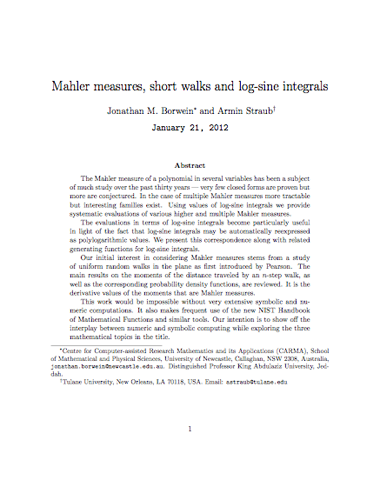#### Measures of Pseudorandomness for Finite Sequences: Minimal Values

N, Alon, Y. Kohayakawa, C. Mauduit, C.G. Moreira and V. Rodl

(Combinatorics, Probability and Computing 15 (1-2) (January 2006), 1—29)

Mauduit and Sárközy introduced and studied certain numerical parameters associated to finite binary sequences EN ∈ {−1, 1} N in order to measure their ‘level of randomness’. Those parameters, the normality measure N (EN), the well-distribution measure W (EN), and the correlation measure Ck(EN) of order k, focus on different combinatorial aspects of EN.

In their work, amongst others, Mauduit and Sárközy (i) investigated the relationship among those parameters and their minimal possible value, (ii) estimated N (EN), W (EN), and Ck(EN) for certain explicitly constructed sequences EN suggested to have a ‘pseudorandom nature’, and (iii) investigated the value of those parameters for genuinely random sequences EN.

In this paper, we continue the work in the direction of (iii) above and determine the order of magnitude of N (EN), W (EN), and Ck(EN) for typical EN. We prove that, for most EN ∈ {−1, 1} N, both W (EN) and N (EN) are of order √ q N, while Ck(EN) is of order N log ` ´ N for k any given 2 ≤ k ≤ N/4.